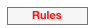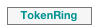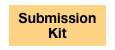April 6, 2011 - a bug was corrected in the BNF for formulae provided in this web site.

Introduction

The objective of this page is to present the BNF (in the ANTLR format) of formula to be provided in the model checking contest. In one file, there can be several formulae, each one referenced by a unique identifier.

You can also access the BNF original file here: mcc-formula.g

There are sets of properties for each model proposed in the submission kit.

BNF (ANTLR format)

rammar formula;

// A formula file is composed of several formulas:

formulas

: formula+ EOF

;

// Each formula has a unique name, a quantifier, and a body.

// It is written <name> = <quantifier> <body>,

// and ends with an end-of-line.

formula

: ID '=' quantifier subformula EOL

;

// A quantifier is either:

// - at least one reachable state satisfies the formula (reachable),

// - all states satisfy the formula (invariant).

quantifier

: 'reachable'

| 'invariant'

;

// The formula body is one of the three cases:

// - an atomic property on the state,

// - a Boolean binary operator (and, or),

// - a Boolean unary operator (not).

subformula

: atomic

| 'not'? '(' subformula ')' (('and' 'not'? '(' subformula ')')+ | ('or' 'not'? '(' subformula ')')+)?

;

// An atomic property is one of the two cases:

// - a particular place of the state contains at least the given tokens,

// - a particular place of the state contains exactly the given tokens.

atomic

: ID 'contains' '{' (token (',' token)*)? '}'

| ID 'equals'   '{' (token (',' token)*)? '}'

;

// A token is composed of its cardinality and its color (value).

token

: cardinality '*' value

;

cardinality

: INT

;

// The color (value) is a nonempty tuple of integers for colored tokens,

// or [.] for black tokens.

value

: '[' ('.' | (INT (',' INT)*) | (ID (',' ID)*) ) ']'

;

ID  :  ('a'..'z'|'A'..'Z'|'_') ('a'..'z'|'A'..'Z'|'0'..'9'|'_')* ;

INT : '0'..'9'+ ;

EOL : '\n' ;

In formulae, AND is evaluated prior to OR as usual.

Some Examples

Please find enclosed an example of file containing two formulas:

f1 = reachable not (p1 contains {1*})

f2 = invariant (p1 contains {1*[.]}) and (p2 equals {1*[1, 2], 3*[2, 1]})

f3 = reachable (p1 equals {}) and (p2 equals {3*[.]})

Formula f1 searches the state where place p1 does not contains at least a token of value 1.

Formula f2 means that for each reachable markings, place p1 contains at least a token with value 1 and place p2 contains exactly 1 token having value [1,2] and 3 tokens having value [2,1].

Formula f3 searches the state where place p1 is empty and place p2 contains 3 tokens (uncolored ones).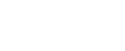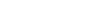Model Checking Contest, BNF for formulae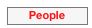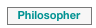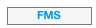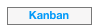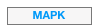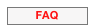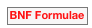Models